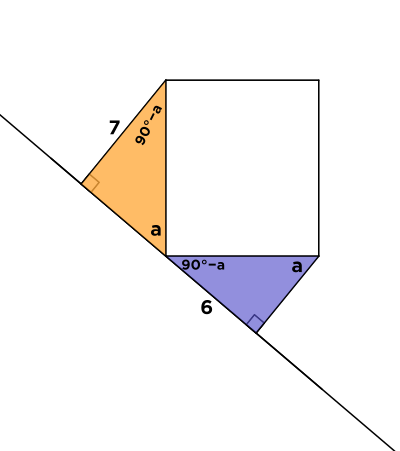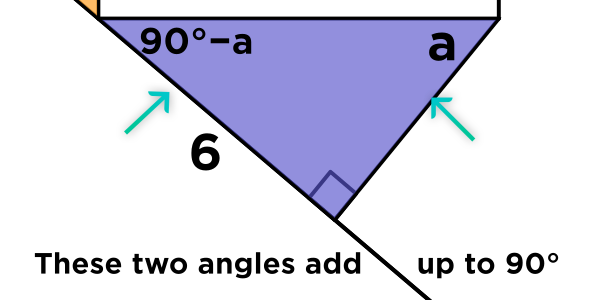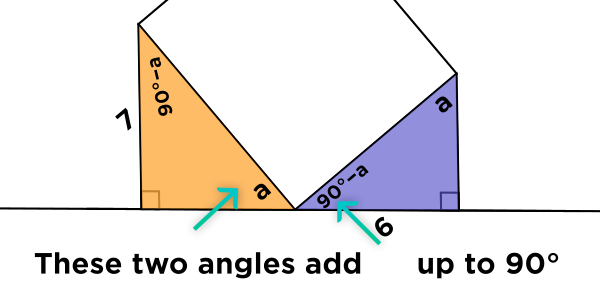# Explanation - How do you know that the angle in the purple triangle is also "a"?

• Module 2 Week 2 Day 5 Challenge Part 5How do you know that the angle in the purple triangle is also "a"?

• @divinedolphin The short answer is that the purple angle \(\angle a\) has measure \(\angle a\) because the other purple angle in the purple triangle has measure \(90^{\circ} - a,\) since the angles of a triangle add up to \(180^{\circ}.\)Then, why is the other purple angle equal to \(90^{\circ} - a?\)

Well, this is because if you start at the yellow angle that is \(\angle a\) and then look at the purple angle that right next to the box corner that is adjacent to it, you can see that these three angles together make up a line:This is because a line has \(180^{\circ}\) degrees, and the right corner accounts for \(90^{\circ}\) of these degrees.

I hope this helps!